Energy

Energy comes in many forms, making the fundamental concept hard to grasp. Energy is conserved: total energy is not created or destroyed, it only changes form. This is true unless we consider fusion or fission processes which occur in places like the core of the Sun. There, matter is transformed into energy and vice versa, following Einstein's formula E = mc2.We all know what it feels like to have "a lot of energy" but how do we define energy in physics? Energy can take several forms, including kinetic energy, potential energy and thermal energy that arises from dissipative forces like friction.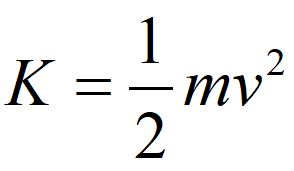Kinetic energy is the energy of motion. Notice is it a scalar quantity. The kinetic energy defined above is translational kinetic energy of a particle, where m is the mass of the particle and v is the velocity of the particle.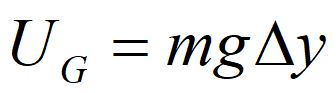Potential energy is energy that is stored in the system. Gravitational potential energy can be converted to kinetic energy.If you do work to lift a massive object in a gravitational field, that energy is stored as potential energy. If you drop the object, the potential energy is converted to kinetic energy as it falls. The farther it falls, the faster it moves (as it accelerates downward) and the more potential energy is converted into kinetic energy.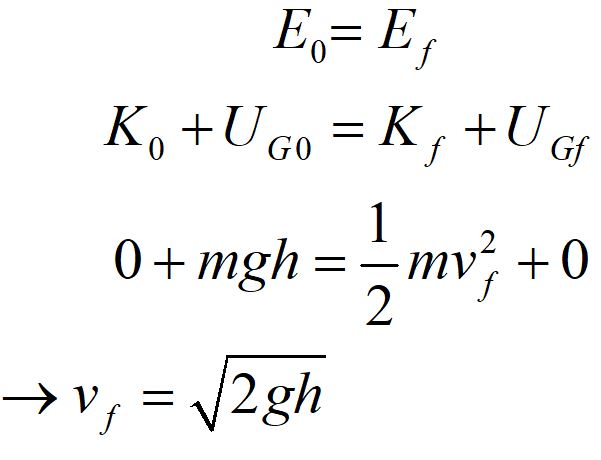Energy is conserved in this situation. Note that the change in potential energy depends on the difference in y. If our gravitational force is constant, we are free to choose the zero of our potential energy. If we lift an object a distance h, hold it at rest and then drop it, we can calculate the final velocity of the object when it returns to its original height.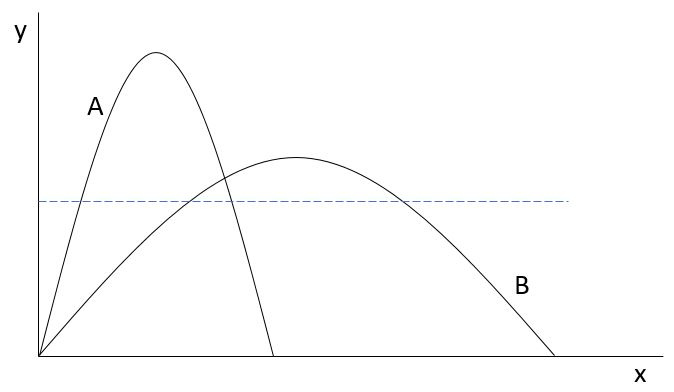Consider the trajectories of a projectile above. If the initial speed was the same for both trajectories, in which trial would the projectile be moving faster when it crossed the dashed line?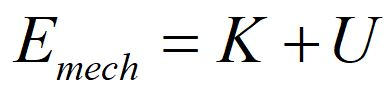Energy that includes just kinetic energy and potential energy is called mechanical energy.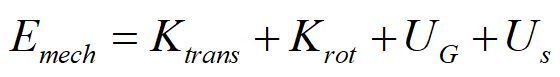Kinetic energy can be translational or rotational. We will only consider translational energy at present. Potential energy can take several forms besides gravitational potential energy, including spring potential energy. Chemical potential energy is another form of potential energy that will not be covered here.

Notice that dissipative energy like friction is not included in mechanical energy. When the only kinds of energy present are kinetic and potential energy, we say that mechanical energy is conserved. Energy can be converted between kinetic and potential, but no energy is dissipated into thermal energy via friction forces.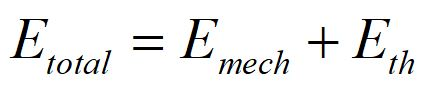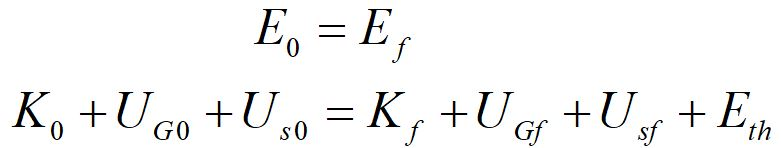Total energy includes mechanical energy and thermal energy. Notice we have included kinetic energy, gravitational and spring potential energy on both the initial side and the final side of the equation, but the thermal energy only appears on the final side of the equation. That is because the thermal energy we are considering arises from friction during the interaction, and is not present beforehand.

There are other forms of energy we will not include here, such as thermal energy of substances. This will be covered in later chapters.

Energy diagramsEnergy diagrams like the one shown above plot mechanical energy as a function of position. Total energy of a particle consists of potential energy plus kinetic energy. The behavior of a particle can be analyzed as the particle moves in a potential. Depending on the total energy available to a particle, it may have points of stable equilibria (dips) unstable equilibria (peaks) and turning points where the kinetic energy goes to zero.

This interactive PhET simulation illustrates concepts of conservation of energy in a simple system of a skateboarder on a track, with and without friction.© 2018 - khadley.com

website developer Kathryn Hadley

web master: jay(at-sign)jirvinh.com